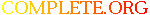Complete.Org: Mailing Lists: Archives: freeciv-ai: November 2002: [freeciv-ai] Re: Measure the AI ability to harvest resources# [freeciv-ai] Re: Measure the AI ability to harvest resources

[Top] [All Lists]

 To: freeciv-ai@xxxxxxxxxxx Subject: [freeciv-ai] Re: Measure the AI ability to harvest resources From: Raimar Falke Date: Mon, 25 Nov 2002 10:41:13 +0100

```On Mon, Nov 25, 2002 at 09:59:26AM +0100, Raimar Falke wrote:
> On Mon, Nov 25, 2002 at 12:48:21AM +0100, Raimar Falke wrote:
> > > 2: 29:            Wang Gon:(f s t)=(  71  147  150) (l t s)=(   0   42
> > > 108) s=  5648 uf=4000
> > > 2: 29:      Guntis Ulmanis:(f s t)=( 123  160  162) (l t s)=(   0   11
> > > 151) s=  7001 uf=4000
> > > 2: 29:   Richard Lionheart:(f s t)=( 108  195   92) (l t s)=(   0   30
> > > 62) s=  6471 uf=4000
> > > 2: 29:             Genghis:(f s t)=( 145  173  139) (l t s)=(   0   19
> > > 120) s=  7364 uf=4000
> >
> > 2: 29:Wang Gon
> > 2:               Settlers: 1 * 40 = 40
> > 2:               Warriors: 1 * 10 = 10
> > 2:               Barracks: 2 * 40 = 80
> > 2:   total = 130 shields
> > 2: 29:Guntis Ulmanis
> > 2:               Settlers: 2 * 40 = 80
> > 2:               Barracks: 1 * 40 = 40
> > 2:   total = 120 shields
> > 2: 29:Richard Lionheart
> > 2:               Settlers: 3 * 40 = 120
> > 2:               Warriors: 1 * 10 = 10
> > 2:               Barracks: 1 * 40 = 40
> > 2:   total = 170 shields
> > 2: 29:Genghis
> > 2:               Settlers: 3 * 40 = 120
> > 2:               Barracks: 1 * 40 = 40
> > 2:   total = 160 shields
>
> Same game after 100 turns (1AD):
>
> 2: 99:            Wang Gon:(f s t)=( 440  856  795) (l t s)=(   0  272  523)
> s= 32452 uf=4000
> 2: 99:      Guntis Ulmanis:(f s t)=(1119 2917 1369) (l t s)=(   0  700  669)
> s= 87278 uf=4000
> 2: 99:   Richard Lionheart:(f s t)=(1271 2974  647) (l t s)=(   0  444  224)
> s= 82471 uf=4000
> 2: 99:             Genghis:(f s t)=(1273 2532  984) (l t s)=(   0  514  430)
> s= 79039 uf=4000
> 2: 99:Wang Gon
> 2:               Settlers: 11 * 40 = 440
> 2:               Warriors: 13 * 10 = 130
> 2:               Barracks: 4 * 40 = 160
> 2:   total = 730 shields
> 2: 99:Guntis Ulmanis
> 2:               Settlers: 19 * 40 = 760
> 2:               Warriors: 1 * 10 = 10
> 2:               Diplomat: 22 * 30 = 660
> 2:               Barracks: 19 * 40 = 760
> 2:             Courthouse: 5 * 80 = 400
> 2:   total = 2590 shields
> 2: 99:Richard Lionheart
> 2:               Settlers: 43 * 40 = 1720
> 2:               Warriors: 1 * 10 = 10
> 2:               Barracks: 20 * 40 = 800
> 2:   total = 2530 shields
> 2: 99:Genghis
> 2:               Settlers: 36 * 40 = 1440
> 2:               Warriors: 17 * 10 = 170
> 2:               Barracks: 17 * 40 = 680
> 2:   total = 2290 shields

Even worst that these shields aren't used to built settlers they also
penalize the AI even further by costing upkeep. Wang Gon and Guntis
Ulmanis both have to set tax rate to 80% to get enough gold. Richard
Lionheart has to sell barracks every third turn even with 100%
tax. Genghis has 100% tax and +-0.

Same game after some more turns (the first player has just finished
building one library) (however the AI doesn't build librarys in any
other city):

2: 165:            Wang Gon:(f s t)=(1465 4391 2069) (l t s)=(   0 1248  821)
s=127310 uf=4000

+1000 tax, +300 bulbs

2: 165:      Guntis Ulmanis:(f s t)=(1748 9580 3830) (l t s)=(  14 2737 1106)
s=242032 uf=4000

+2000 tax, +400 bulbs

2: 165:   Richard Lionheart:(f s t)=(1522 6348 1200) (l t s)=(   0  997  299)
s=151234 uf=4000

+500 tax, +75 bulbs !!!!!!!

2: 165:             Genghis:(f s t)=(1502 5160 2080) (l t s)=(  15 1493  447)
s=141218 uf=4000

+1000 tax, +17 bulbs !!!!!!!

2: 165:Wang Gon
2:               Settlers: 43 * 40 = 1720
2:               Warriors: 16 * 10 = 160
2:               Barracks: 19 * 40 = 760
2:             Courthouse: 11 * 80 = 880
2:   total = 3520 shields
2: 165:Guntis Ulmanis
2:               Settlers: 22 * 40 = 880
2:               Warriors: 1 * 10 = 10
2:               Diplomat: 217 * 30 = 6510
2:               Barracks: 20 * 40 = 800
2:             Courthouse: 13 * 80 = 1040
2:                Library: 1 * 80 = 80
2:   total = 9320 shields
2: 165:Richard Lionheart
2:               Settlers: 64 * 40 = 2560
2:               Warriors: 1 * 10 = 10
2:               Barracks: 36 * 40 = 1440
2:   total = 4010 shields
2: 165:Genghis
2:               Settlers: 52 * 40 = 2080
2:               Warriors: 29 * 10 = 290
2:               Barracks: 22 * 40 = 880
2:   total = 3250 shields

Raimar

--
email: rf13@xxxxxxxxxxxxxxxxx
Windows: From the people who brought you edlin...

```